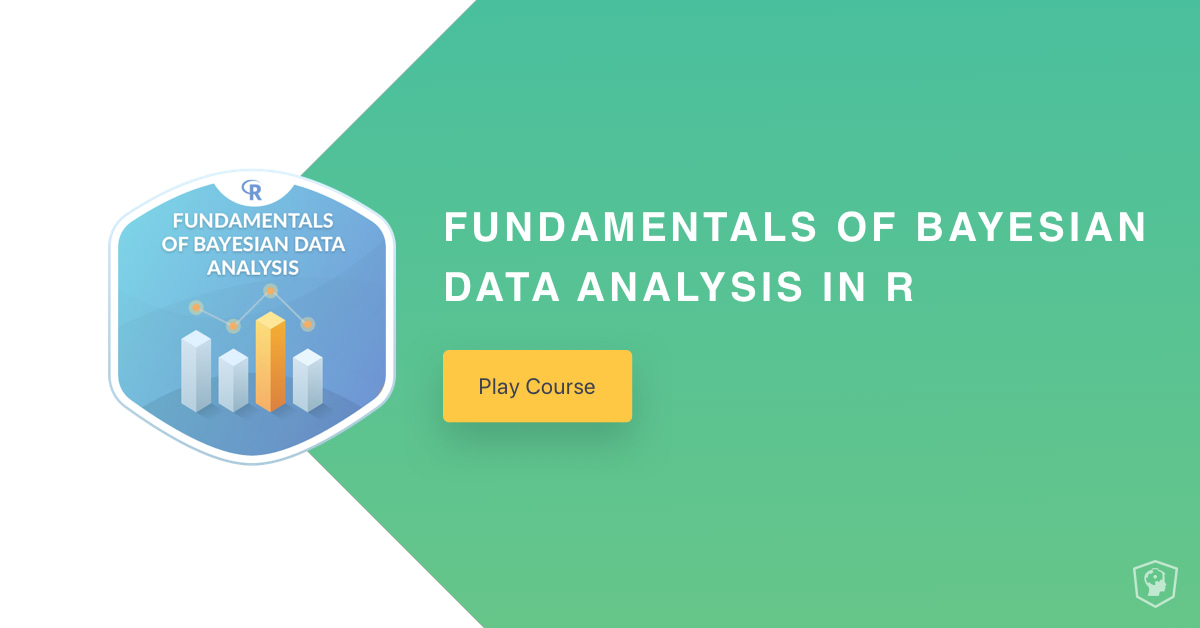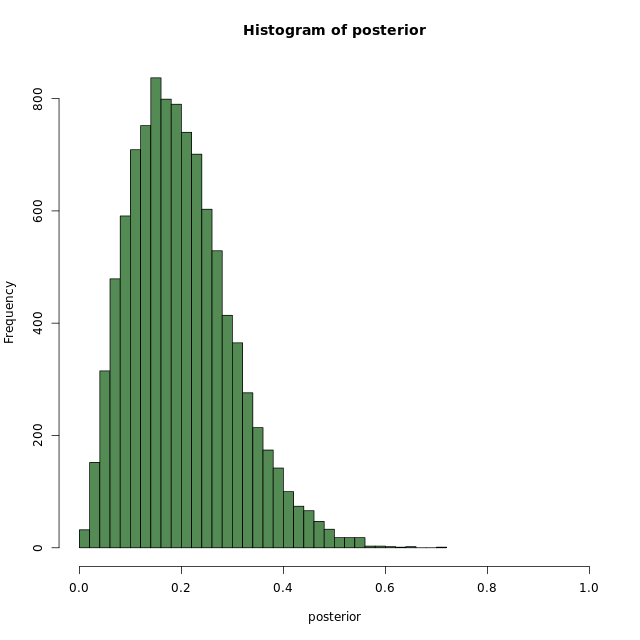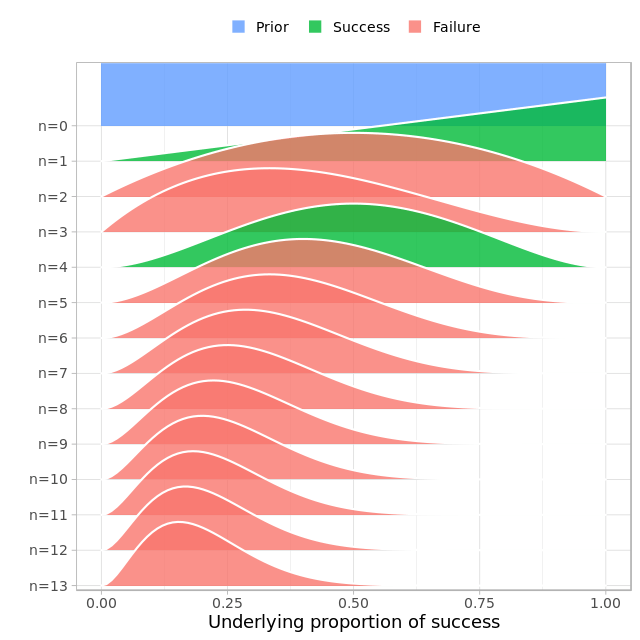# New Course: Fundamentals of Bayesian Data Analysis in R

Learn about our new R course.
Aug 2018  · 2 min readHere is the course link.

## Course Description

Bayesian data analysis is an approach to statistical modeling and machine learning that is becoming more and more popular. It provides a uniform framework to build problem specific models that can be used for both statistical inference and for prediction. This course will introduce you to Bayesian data analysis: What it is, how it works, and why it is a useful tool to have in your data science toolbox.

## Chapter 1: What is Bayesian Data Analysis? (Free)

This chapter will introduce you to Bayesian data analysis and give you a feel for how it works.## Chapter 2: How does Bayesian inference work?

In this chapter we will take a detailed look at the foundations of Bayesian inference.## Chapter 3: Why use Bayesian Data Analysis?

This chapter will show you four reasons why Bayesian data analysis is a useful tool to have in your data science tool belt.## Chapter 4: Bayesian inference with Bayes' theorem

Learn what Bayes theorem is all about and how to use it for statistical inference.## Chapter 5: More parameters, more data, and more Bayes

Learn about using the Normal distribution to analyze continuous data and try out a tool for practical Bayesian analysis in R.